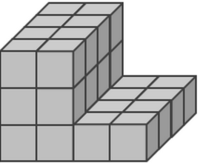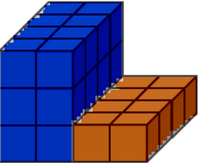# Breaking Apart Composite Solids

Alignments to Content Standards: 5.MD.C.5 5.MD.C.5.c

Students will need two different color markers or crayons to complete this task.

John was finding the volume of this figure. He decided to break it apart into two separate rectangular prisms. John found the volume of the solid below using this expression: (4 x 4 x 1) + (2 x 4 x 2).

Decompose the figure into two rectangular prisms and shade them in different colors to show one way John might have thought about it.Phillis also broke this solid into two rectangular prisms, but she did it differently than John.  She found the volume of the solid below using this expression: (2 x 4 x 3) + (2 x 4 x 1).

Decompose the figure into two rectangular prisms and shade them in different colors to show one way Phillis might have thought about it.## IM Commentary

The purpose of this task is to help students see different ways of breaking a solid into right rectangular prisms and to relate volume to the operations of multiplication and division. This task combines the work that students have done in the Operations and Algebraic Thinking domain with their work on volume in the Measurement and Data domain. This task requires students to think backwards from two different given expressions to understand how different students might decompose the same irregular solid.

There are a number of possible extensions to this task. Teachers could find similar irregular solids and ask students to create different expressions to show different ways of decomposing the solids. Teachers could also pose slightly more complex expressions for this problem such as:

• 4 x (3 x 2 + 2) or
• (4 x 4 x 3) - (2 x 4 x 2)

In the first example, it is very helpful to build the shape with cubes so that students can see how this solid might be broken apart. Similarly, the second example involves picturing a larger solid which might be helpful to build out with blocks. Students would then understand why a number is being subtracted out at the end.

This task engages students in looking for and making use of structure (MP 7) at the fifth grade level.  As students break apart the shape into different pieces they are seeing structure in the figure, and they are making use of that structure by adding the volumes of the pieces to find the volume of the entire shape.

## Solution

John's picture could be:Phillis' picture could be: